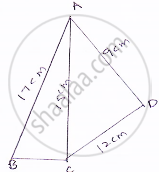Share

Books Shortlist

# Find the Perimeter and Area of the Quadrilateral Abcd in Which Ab = 17 Cm, Ad =9 Cm, Cd = L2cm, ∠Acb = 90° and Ac=L5cm. - CBSE Class 9 - Mathematics

ConceptApplication of Heron’S Formula in Finding Areas of Quadrilaterals

#### Question

Find the perimeter and area of the quadrilateral ABCD in which AB = 17 cm, AD =9 cm, CD = l2cm, ∠ACB = 90° and AC=l5cm.

#### Solution

The sides of a quadrilateral ABCD in which AB = 17 cm, AD = 9 cm, CD = 12 cm, ∠ACB = 90° and AC = 15 cm
Here, By using Pythagoras theorem

BC=sqrt(17^2-15^2) = sqrt(289-225)=sqrt64=8cm

Now, area of ΔABC=1/2xx8xx15=60cm^2

For area of Δle ACD,
Let a = 15 cm, b = 12 cm and c = 9 cm

Therefore, S =(15+12+9)/2=36/2=18cmArea of ACD =sqrt(s(s-a)(s-b)(s-c))

=sqrt(18(18-15)(18-12)(18-9))

=sqrt(18xx18xx3xx3)

=sqrt((18xx3)^2)

=54cm^2

∴ Thus, the area of quadrilateral ABCD = 60 + 54 = 114 cm^2

Is there an error in this question or solution?

#### APPEARS IN

Solution Find the Perimeter and Area of the Quadrilateral Abcd in Which Ab = 17 Cm, Ad =9 Cm, Cd = L2cm, ∠Acb = 90° and Ac=L5cm. Concept: Application of Heron’S Formula in Finding Areas of Quadrilaterals.
S